#### 期刊菜单

Thermal and Chemical Shock Dynamic Response of a Semiinfinite Thin Plate Considering Scale Effect and Memory Dependent Effect

Abstract: Based on memory-dependent differential theory, the dynamic response of semi-infinite thin plates subjected to thermal and chemical shocks is studied. In this paper, the generalized thermoelastic governing equation of semi-infinite thin plate considering the scale effect and memory dependent effect is given. The governing equation is solved by the Laplace integral transform and its numerical inverse transform. The equations of semi-infinite thin plate under thermal and chemical shock are obtained. Influence of non-local parameters, heat conduction time hysteresis factor and diffusion time hysteresis factor on temperature, stress, displacement, concentration and chemical potential. The results show that the non-local parameters have little effect on temperature, concentration and chemical potential. Kernel function selection which embodies memory effect has different influences on each physical quantity.

1. 引言

Qi and Suh  在2010年提出了广义热弹动力学理论，是一种适用于超快激光脉冲激励下硅薄膜结构热力耦合响应研究的广义热弹动力学公式；Kuang  提出了惯性熵热弹性理论，提出的非线性介电通用热力学变异原理，将其延伸至热电，作为热电通化动力学理论的基本物理原理。Wang和Zhang  提出了热质量热弹性理论，他从经典热传导定律出发，热质量被定义为电介质中声子气体的等效质量并考虑了惯性效应，推导并给出了各向异性非均匀材料和各向同性均匀材料的计算公式，证明了各向同性均质材料方程组的唯一性定理。与其它广义热弹性理论相比，基于热质量运动的理论更能合理地预测微尺度热传导条件下热弹性波的传播。

Sherief  等利用分数阶微积分的方法推导出一个新的热弹性理论，即耦合热弹性理论和具有一个弛豫时间的广义热弹性理论作为极限情况，该理论允许热波以有限的速度传播，他是在L-S理论的基础上加入Caputo型分数阶导数；Sherief和Saleh  考虑了渗透物质接触的边界平面下热弹性半空间的问题，广义热弹性扩散理论与一个松弛时间，并利用基于拉普拉斯反变换的方法，数值求解了物理域中的问题，得到了温度、位移、应力、浓度和化学势；马永斌等  分数阶广义热弹性理论的基础上，对球型空腔的无限大体受热冲击时的动态响应做了研究；何天虎等  在Sherief分数阶热弹性理论基础上对无限长圆柱导体模型的动态响应问题进行了研究。

Caputo分数阶微分具有描述系统或物理过程的瞬时变化率受过去情形的影响的优点，但是不能很好地反映出当时间t比较大时的记忆影响。因此，Wang和Li  创造性地提出了“记忆依赖微分”这一概念，它的优点在于可以选取不同的核函数来反映不同的记忆影响；Ezzat  等提出了一种基于泰勒级数展开的弹性热扩散数学模型，根据数值结果建立了记忆依赖微分的影响；李妍  基于记忆依赖微分的广义热弹扩散问题的动态响应问题做了研究。

2. 基本方程

${\sigma }_{ij,j}=\rho {\stackrel{¨}{u}}_{i}$ (1)

${e}_{ij}=\frac{1}{2}\left({\stackrel{¨}{u}}_{i,j}+{\stackrel{¨}{u}}_{j,i}\right)$ (2)

${\sigma }_{ij}=2\mu {e}_{i,j}+\left[{\tau }_{0}{e}_{kk}-{r}_{1}\theta -{r}_{2}P\right]{\delta }_{ij},\text{\hspace{0.17em}}\text{\hspace{0.17em}}i,j=1,2,3$ (3)

$\left(1+{\tau }_{1}{D}_{{\tau }_{1}}\right){q}_{i}=-{k}_{ij}{\theta }_{,j}$ (4)

$\left(1+{\tau }_{2}{D}_{{\tau }_{2}}\right){\eta }_{i}=-{\Gamma }_{ij}{P}_{,j}$ (5)

${q}_{i,i}=-\rho {T}_{0}\stackrel{˙}{S}$ (6)

$\rho S={r}_{1}{e}_{kk}+{l}_{1}\theta +dP$ (7)

${r}_{1}={\beta }_{1}+\frac{a}{b}{\beta }_{2},\text{\hspace{0.17em}}{l}_{1}=\frac{\rho {C}_{E}}{{T}_{0}}+\frac{{a}^{2}}{b},\text{\hspace{0.17em}}d=\frac{a}{b},\text{\hspace{0.17em}}{\beta }_{1}=\left(3\lambda +2\mu \right){\alpha }_{t},\text{\hspace{0.17em}}{\beta }_{2}=\left(3\lambda +2\mu \right){\alpha }_{c}$

${\alpha }_{t}$ 是材料的线性热膨胀系数， ${\alpha }_{c}$ 是线性扩散膨胀系数。

${\eta }_{i,i}=-\stackrel{˙}{C}$ (8)

$C={r}_{2}{e}_{kk}+d\theta +nP$ (9)

${\sigma }_{ij}\left[1-{\left({e}_{0}a\right)}^{2}{\nabla }^{2}\right]=2\mu {e}_{i,j}+\left[{\lambda }_{0}{e}_{kk}-{r}_{1}\theta -{r}_{2}P\right]{\delta }_{ij}$ (10)

$\rho {\stackrel{˙}{u}}_{i}\left[1-{\left({e}_{0}a\right)}^{2}{\nabla }^{2}\right]=\mu {u}_{i,jj}+\left[\left({\lambda }_{0}+\mu \right){u}_{j,ij}-{r}_{1}{\theta }_{,i}-{r}_{2}{P}_{,i}\right]$ (11)

$\left(1+{\tau }_{1}{D}_{{\tau }_{1}}\right)\left({T}_{0}{r}_{1}{\stackrel{˙}{e}}_{kk}+{T}_{0}{l}_{1}\stackrel{˙}{\theta }+{T}_{0}d\stackrel{˙}{P}\right)=k{\theta }_{,jj}$ (12)

$\left(1+{\tau }_{2}{D}_{{\tau }_{2}}\right)\left({r}_{2}{\stackrel{˙}{e}}_{kk}+d\stackrel{˙}{\theta }+n\stackrel{˙}{P}\right)=\Gamma {P}_{,jj}$ (13)

3. 问题描述

${u}_{x}=u\left(x,t\right),{u}_{y}={u}_{z}=0$ (14)

$e={e}_{kk}=\frac{\partial u}{\partial t}$ (15)

$\sigma \left[1-{\left({e}_{0}a\right)}^{2}{\nabla }^{2}\right]=\left(2\mu +{\lambda }_{0}\right)\frac{\partial u}{\partial t}-{r}_{1}\theta -{r}_{2}P$ (16)

$\rho \frac{{\partial }^{2}u}{\partial {t}^{2}}\left[1-{\left({e}_{0}a\right)}^{2}{\nabla }^{2}\right]=\left({\lambda }_{0}+2\mu \right)\frac{{\partial }^{2}u}{\partial {x}^{2}}-{r}_{1}\frac{\partial \theta }{xx}-{r}_{2}\frac{\partial P}{\partial x}$ (17)

$\left(1+{\tau }_{1}{D}_{{\tau }_{1}}\right)\left({T}_{0}{r}_{1}\frac{{\partial }^{2}u}{\partial x\partial t}+{T}_{0}{l}_{1}\frac{\partial \theta }{\partial t}+{T}_{0}d\frac{\partial P}{\partial t}\right)=k\frac{{\partial }^{2}\theta }{\partial {x}^{2}}$ (18)

$\left(1+{\tau }_{2}{D}_{{\tau }_{2}}\right)\left({r}_{2}\frac{{\partial }^{2}u}{\partial x\partial t}+d\frac{\partial \theta }{\partial t}+n\frac{\partial P}{\partial t}\right)=\Gamma \frac{{\partial }^{2}P}{\partial {x}^{2}}$ (19)

$\begin{array}{l}{x}^{\prime }={c}_{1}\eta x,\text{\hspace{0.17em}}{u}^{\prime }={c}_{1}\eta u,\text{\hspace{0.17em}}{\left({e}_{0}a\right)}^{\prime }={c}_{1}\eta {e}_{0}a,\text{\hspace{0.17em}}{t}^{\prime }={c}_{1}^{2}\eta t,\text{\hspace{0.17em}}{\theta }^{\prime }=\frac{{\beta }_{1}\theta }{\rho {c}_{1}^{2}},\text{\hspace{0.17em}}{P}^{\prime }=\frac{P}{{\beta }_{2}},\\ {C}^{\prime }=\frac{{\beta }_{2}C}{\rho {c}_{1}^{2}},\text{\hspace{0.17em}}{\sigma }^{\prime }=\frac{\sigma }{\rho {c}_{1}^{2}},\text{\hspace{0.17em}}{q}^{\prime }=\frac{q}{k{T}_{0}{c}_{1}\eta },\text{\hspace{0.17em}}{{L}^{\prime }}_{0}=\frac{{c}_{1}\eta {L}_{0}}{{T}_{0}{C}_{E}\rho },\text{\hspace{0.17em}}{c}_{1}^{2}=\frac{\lambda +2\mu }{\rho },\text{\hspace{0.17em}}\eta =\frac{{C}_{E}\rho }{k}\end{array}$

$\sigma \left[1-{\left({e}_{0}a\right)}^{2}\frac{{\partial }^{2}}{\partial {x}^{2}}\right]={\alpha }_{1}\frac{\partial u}{\partial x}-{\alpha }_{2}\theta -{\alpha }_{3}P,$ (20)

$\frac{{\partial }^{2}u}{\partial {t}^{2}}\left[1-{\left({e}_{0}a\right)}^{2}\frac{{\partial }^{2}}{\partial {x}^{2}}\right]{\epsilon }_{1}=\frac{{\partial }^{2}u}{\partial {x}^{2}}-{\epsilon }_{2}\frac{\partial \theta }{\partial x}-{\epsilon }_{3}\frac{\partial P}{\partial x},$ (21)

$\left(1+{\tau }_{1}{D}_{{\tau }_{1}}\right)\left({\psi }_{1}\frac{{\partial }^{2}u}{\partial x\partial t}+{\psi }_{2}\frac{\partial \theta }{\partial t}+{\psi }_{3}\frac{\partial P}{\partial t}\right)=\frac{{\partial }^{2}\theta }{\partial {x}^{2}},$ (22)

$\left(1+{\tau }_{2}{D}_{{\tau }_{2}}\right)\left({\gamma }_{1}\frac{{\partial }^{2}u}{\partial x\partial t}+{\gamma }_{2}\frac{\partial \theta }{\partial t}+{\gamma }_{3}\frac{\partial P}{\partial t}\right)=\frac{{\partial }^{2}P}{\partial {x}^{2}},$ (23)

$C={\phi }_{1}\frac{\partial u}{\partial t}+{\phi }_{2}\theta +{\phi }_{3}P,$ (24)

$\begin{array}{l}{\alpha }_{1}=\frac{{\lambda }_{0}+2\mu }{\lambda +2\mu },\text{\hspace{0.17em}}{\alpha }_{2}=\frac{{r}_{1}}{{\beta }_{1}},\text{\hspace{0.17em}}{\alpha }_{3}=\frac{{r}_{2}+{\beta }_{2}}{\lambda +2\mu },\text{\hspace{0.17em}}{\epsilon }_{1}=\frac{1}{{\alpha }_{1}},\text{\hspace{0.17em}}{\epsilon }_{2}=\frac{{r}_{1}}{{\alpha }_{1}{\beta }_{1}},\text{\hspace{0.17em}}{\epsilon }_{3}=\frac{{r}_{2}{\beta }_{2}}{{\lambda }_{0}+2\mu },\text{\hspace{0.17em}}{\psi }_{1}=\frac{{T}_{0}{r}_{1}{\beta }_{1}}{k\rho {c}_{1}^{2}\eta },\\ {\psi }_{2}=\frac{{T}_{0}{l}_{1}}{k\eta },\text{\hspace{0.17em}}{\psi }_{3}=\frac{{T}_{0}d{\beta }_{1}{\beta }_{2}}{k\rho {c}_{1}^{2}\eta },\text{\hspace{0.17em}}{\gamma }_{1}=\frac{{r}_{2}}{\Gamma \eta {\beta }_{2}},\text{\hspace{0.17em}}{\gamma }_{2}=\frac{d{c}_{1}^{2}\rho }{\Gamma \eta {\beta }_{1}{\beta }_{2}},\text{\hspace{0.17em}}{\gamma }_{3}=\frac{n}{\Gamma \eta },\text{\hspace{0.17em}}{\phi }_{1}=\frac{{r}_{2}{\beta }_{2}}{\rho {c}_{1}^{2}},\text{\hspace{0.17em}}{\phi }_{2}=\frac{d{\beta }_{2}}{{\beta }_{1}},\text{\hspace{0.17em}}{\phi }_{3}=\frac{n{\beta }_{2}^{2}}{\rho {c}_{1}^{2}}\end{array}$

$u\left(x,0\right)=\theta \left(x,0\right)=C\left(x,0\right)=0,t>0;$

$\stackrel{˙}{u}\left(x,0\right)=\stackrel{˙}{\theta }\left(x,0\right)=\stackrel{˙}{C}\left(x,0\right)=0,t>0;$

$\stackrel{¨}{u}\left(x,0\right)=\stackrel{¨}{\theta }\left(x,0\right)=\stackrel{¨}{C}\left(x,0\right)=0,t>0;$

$u\left(x,t\right),\theta \left(x,t\right),C\left(x,t\right)\to 0$

$k\left(t-\xi \right)=\left\{\begin{array}{ll}1,\hfill & 当\text{ }\text{ }p=q=0,\hfill \\ 1-\frac{\left(\tau -\xi \right)}{\tau },\hfill & 当\text{ }\text{ }p=0,q=\frac{1}{2},\hfill \\ 1-\left(\tau -\xi \right),\hfill & 当\text{ }\text{ }p=0,q=\frac{\tau }{2},\hfill \end{array}$

4. 拉普拉斯变换

$\stackrel{¯}{f}\left(x,s\right)=L\left[f\left(x,t\right)\right]={\int }_{0}^{\infty }{\text{e}}^{-st}f\left(x,t\right)\text{d}t,\mathrm{Re}\left(s\right)>0$ (25)

$\stackrel{¯}{\sigma }\left[1-{\left({e}_{0}a\right)}^{2}\frac{{\text{d}}^{2}}{\text{d}{x}^{2}}\right]={\alpha }_{1}\frac{\text{d}\stackrel{¯}{u}}{\text{d}x}-{\alpha }_{2}\stackrel{¯}{\theta }-{\alpha }_{3}\stackrel{¯}{P},$ (26)

$\frac{{\text{d}}^{2}\stackrel{¯}{u}}{\text{d}{t}^{2}}\left[1-{\left({e}_{0}a\right)}^{2}\frac{{\text{d}}^{2}}{\text{d}{x}^{2}}\right]{\epsilon }_{1}=\frac{{\text{d}}^{2}\stackrel{¯}{u}}{\text{d}{x}^{2}}-{\epsilon }_{2}\frac{\text{d}\stackrel{¯}{\theta }}{\text{d}x}-{\epsilon }_{3}\frac{\text{d}\stackrel{¯}{P}}{\text{d}x},$ (27)

$s\left(1+{G}_{1}\right)\left({\psi }_{1}\frac{{\text{d}}^{2}\stackrel{¯}{u}}{\text{d}x}+{\psi }_{2}\stackrel{¯}{\theta }+{\psi }_{3}\stackrel{¯}{P}\right)=\frac{{\partial }^{2}\stackrel{¯}{\theta }}{\partial {x}^{2}},$ (28)

$\left(1+{G}_{2}\right)\left({\gamma }_{1}\frac{\text{d}\stackrel{¯}{u}}{\text{d}x}+{\gamma }_{2}\stackrel{¯}{\theta }+{\gamma }_{3}\stackrel{¯}{P}\right)=\frac{{\text{d}}^{2}\stackrel{¯}{P}}{\text{d}{x}^{2}},$ (29)

$\stackrel{¯}{C}={\phi }_{1}\frac{\text{d}\stackrel{¯}{u}}{\text{d}t}+{\phi }_{2}\stackrel{¯}{\theta }+{\phi }_{3}\stackrel{¯}{P},$ (30)

$L\left[\left({\psi }_{1}\frac{{\partial }^{2}u}{\partial x\partial t}+{\psi }_{2}\frac{\partial \theta }{\partial t}+{\psi }_{3}\frac{\partial P}{\partial t}\right)\right]=\left({\psi }_{1}\frac{{\text{d}}^{2}\stackrel{¯}{u}}{\text{d}x}+{\psi }_{2}\stackrel{¯}{\theta }+{\psi }_{3}\stackrel{¯}{P}\right)$

$L\left[\left({\gamma }_{1}\frac{{\partial }^{2}u}{\partial x\partial t}+{\gamma }_{2}\frac{\partial \theta }{\partial t}+{\gamma }_{3}\frac{\partial P}{\partial t}\right)\right]=\left({\gamma }_{1}\frac{\text{d}\stackrel{¯}{u}}{\text{d}x}+{\gamma }_{2}\stackrel{¯}{\theta }+{\gamma }_{3}\stackrel{¯}{P}\right);$

${G}_{1}=1-\frac{2b}{s{\tau }_{1}}+\frac{2{a}^{2}}{s{\tau }_{1}^{2}}-{\text{e}}^{-s{\tau }_{1}}\left[\left(1-2b+{a}^{2}\right)+\frac{2\left({a}^{2}-b\right)}{s{\tau }_{1}}+\frac{2{a}^{2}}{{s}^{2}{\tau }_{1}^{2}}\right]$ (31)

${G}_{2}=1-\frac{2b}{s{\tau }_{2}}+\frac{2{a}^{2}}{s{\tau }_{2}^{2}}-{\text{e}}^{-s{\tau }_{2}}\left[\left(1-2b+{a}^{2}\right)+\frac{2\left({a}^{2}-b\right)}{s{\tau }_{2}}+\frac{2{a}^{2}}{{s}^{2}{\tau }_{2}^{2}}\right]$ (32)

$L\left[{\tau }_{1}{D}_{{\tau }_{1}}\right]=\left\{\begin{array}{l}1-{\text{e}}^{-s{\tau }_{1}},k\left(t-\xi \right)=1\\ 1-\frac{1}{s{\tau }_{1}}\left(1-{\text{e}}^{-s{\tau }_{1}}\right),k\left(t-\xi \right)=1-\frac{\left(t-\xi \right)}{\tau }\\ \left(1-{\text{e}}^{-s{\tau }_{1}}\right)-\frac{1}{s{\tau }_{1}}\left(1-{\text{e}}^{-s{\tau }_{1}}\right)+{\tau }_{1}{\text{e}}^{-s{\tau }_{1}},k\left(t-\xi \right)=1-\left(t-\xi \right)\end{array}$

$L\left[{\tau }_{2}{D}_{{\tau }_{2}}\right]=\left\{\begin{array}{l}1-{\text{e}}^{-s{\tau }_{2}},k\left(t-\xi \right)=1\\ 1-\frac{1}{s{\tau }_{2}}\left(1-{\text{e}}^{-s{\tau }_{2}}\right),k\left(t-\xi \right)=1-\frac{\left(t-\xi \right)}{\tau }\\ \left(1-{\text{e}}^{-s{\tau }_{2}}\right)-\frac{1}{s{\tau }_{2}}\left(1-{\text{e}}^{-s{\tau }_{2}}\right)+{\tau }_{2}{\text{e}}^{-s{\tau }_{2}},k\left(t-\xi \right)=1-\left(t-\xi \right)\end{array}$

$\frac{{\text{d}}^{6}}{\text{d}{x}^{6}}\stackrel{¯}{U}-L\frac{{\text{d}}^{4}}{\text{d}{x}^{4}}\stackrel{¯}{U}+M\frac{{\text{d}}^{2}}{\text{d}{x}^{2}}\stackrel{¯}{U}-N\stackrel{¯}{U}=0$ (33)

$L=\frac{{\epsilon }_{1}{s}^{2}+s\left(1+{G}_{1}\right)\left({\psi }_{2}g+{\epsilon }_{2}{\psi }_{1}\right)+s\left(1+{G}_{2}\right)\left({\gamma }_{3}g+{\epsilon }_{3}{\gamma }_{1}\right)}{g}$

$M=\frac{{s}^{2}\left(1+{G}_{1}\right)\left(1+{G}_{2}\right)\left[g\left({\psi }_{2}{\gamma }_{3}-{\psi }_{3}{\gamma }_{2}\right)+{\epsilon }_{1}\left({\psi }_{2}{\gamma }_{1}-{\psi }_{1}{\gamma }_{2}\right)\right]+{\epsilon }_{2}\left[s\left(1+{G}_{1}\right){\psi }_{2}+s\left(1+{G}_{2}\right){\gamma }_{3}\right]}{g}$

$N=\frac{{\epsilon }_{1}{s}^{4}\left(1+{G}_{1}\right)\left(1+{G}_{2}\right)\left[\left({\psi }_{2}{\gamma }_{3}-{\psi }_{3}{\gamma }_{2}\right)\right]}{g},\text{\hspace{0.17em}}\text{\hspace{0.17em}}g=1+{\epsilon }_{1}{s}^{2}{\left({e}_{0}a\right)}^{2}$

$\frac{{\text{d}}^{6}}{\text{d}{x}^{6}}\stackrel{¯}{P}-L\frac{{\text{d}}^{4}}{\text{d}{x}^{4}}\stackrel{¯}{P}+M\frac{{\text{d}}^{2}}{\text{d}{x}^{2}}\stackrel{¯}{P}-N\stackrel{¯}{P}=0$ (34)

$\frac{{\text{d}}^{6}}{\text{d}{x}^{6}}\stackrel{¯}{\theta }-L\frac{{\text{d}}^{4}}{\text{d}{x}^{4}}\stackrel{¯}{\theta }+M\frac{{\text{d}}^{2}}{\text{d}{x}^{2}}\stackrel{¯}{\theta }-N\stackrel{¯}{\theta }=0$ (35)

$\stackrel{¯}{U}\left(x,s\right)=\underset{i=1}{\overset{3}{\sum }}{\stackrel{¯}{U}}_{i}{\text{e}}^{-{k}_{i}x}$ (36)

$\stackrel{¯}{\theta }\left(x,s\right)=\underset{i=1}{\overset{3}{\sum }}{p}_{i}{\stackrel{¯}{U}}_{i}{\text{e}}^{-{k}_{i}x}$ (37)

$\stackrel{¯}{P}\left(x,s\right)=\underset{i=1}{\overset{3}{\sum }}{q}_{i}{\stackrel{¯}{U}}_{i}{\text{e}}^{-{k}_{i}x}$ (38)

${p}_{i}=\frac{-{k}_{i}\left[{\psi }_{3}{\gamma }_{1}{s}^{2}\left(1+{G}_{1}\right)\left(1+{G}_{2}\right)+{\psi }_{1}s\left(1+{G}_{1}\right)\left({k}_{i}^{2}-{\gamma }_{3}s\left(1+{G}_{2}\right)\right)\right]}{{k}_{i}^{2}-{\psi }_{2}s\left(1+{G}_{1}\right)}$

${q}_{i}=\frac{-{k}_{i}\left[{\gamma }_{1}s\left(1+{G}_{2}\right)\left({k}_{i}^{2}-{\psi }_{2}s\left(1+{G}_{1}\right)\right)+{\psi }_{1}{\gamma }_{2}{s}^{2}\left(1+{G}_{1}\right)\left(1+{G}_{2}\right)\right]}{{k}_{i}^{2}-{\psi }_{2}s\left(1+{G}_{1}\right)}$

${k}_{i}$ 是上述方程的特征方程的三个根为

${k}^{6}-L{k}^{4}+M{k}^{2}-N=0$ (39)

${k}_{1}=\sqrt{\frac{1}{3}\left(2\Omega \mathrm{sin}Q+L\right)}$ (40)

${k}_{2}=\sqrt{-\frac{\Omega }{3}\left(\sqrt{3}\mathrm{cos}Q+\mathrm{sin}Q\right)+\frac{L}{3}}$ (41)

${k}_{3}=\sqrt{\frac{\Omega }{3}\left(\sqrt{3}\mathrm{cos}Q-\mathrm{sin}Q\right)+\frac{L}{3}}$ (42)

$\stackrel{¯}{\sigma }\left(x,s\right)=\underset{i=1}{\overset{3}{\sum }}{H}_{i}{\stackrel{¯}{U}}_{i}{\text{e}}^{-{k}_{i}x}$ (43)

$\stackrel{¯}{C}\left(x,s\right)=\underset{i=1}{\overset{3}{\sum }}{O}_{i}{\stackrel{¯}{U}}_{i}{\text{e}}^{-{k}_{i}x}$ (44)

${H}_{i}=\frac{-\frac{1}{{\epsilon }_{1}}{k}_{i}-\frac{{r}_{1}}{{\beta }_{1}}{p}_{i}-\frac{{r}_{2}}{\mu }{q}_{i}}{1-{\left({e}_{0}a\right)}^{2}{k}_{i}^{2}},{O}_{i}=\frac{{r}_{2}}{\mu }\left({q}_{i}-{k}_{i}\right)+\frac{{\alpha }_{c}}{{\alpha }_{t}}d{p}_{i}$

5. 边界条件

${\theta }_{\left(0,t\right)}={f}_{0}{H}_{\left(t\right)},{\sigma }_{\left(0,t\right)}=0,{P}_{\left(0,t\right)}={\varphi }_{0}{H}_{\left(t\right)}$ (45)

${\stackrel{¯}{\theta }}_{\left(0,s\right)}=\frac{{f}_{0}}{s},{\stackrel{¯}{\sigma }}_{\left(0,s\right)}=0,{\stackrel{¯}{P}}_{\left(0,s\right)}=\frac{{\varphi }_{0}}{s}$ (46)

${u}_{1}=\frac{-\frac{1}{s}\left({d}_{2}{q}_{3}-{d}_{3}{q}_{2}\right)+\frac{1}{s}\left({d}_{2}{p}_{3}-{d}_{3}{p}_{2}\right)}{{d}_{1}\left({p}_{2}{q}_{3}-{p}_{3}{q}_{2}\right)+{d}_{2}\left({p}_{3}{q}_{1}-{p}_{1}{q}_{3}\right)+{d}_{3}\left({p}_{1}{q}_{2}-{p}_{2}{q}_{1}\right)}$ (47)

${u}_{2}=\frac{\frac{1}{s}\left({d}_{1}{q}_{3}-{d}_{3}{q}_{1}\right)-\frac{1}{s}\left({d}_{1}{p}_{3}-{d}_{3}{p}_{1}\right)}{{d}_{1}\left({p}_{2}{q}_{3}-{p}_{3}{q}_{2}\right)+{d}_{2}\left({p}_{3}{q}_{1}-{p}_{1}{q}_{3}\right)+{d}_{3}\left({p}_{1}{q}_{2}-{p}_{2}{q}_{1}\right)}$ (48)

${u}_{3}=\frac{-\frac{1}{s}\left({d}_{1}{q}_{2}-{d}_{2}{q}_{1}\right)+\frac{1}{s}\left({d}_{1}{p}_{2}-{d}_{2}{p}_{1}\right)}{{d}_{1}\left({p}_{2}{q}_{3}-{p}_{3}{q}_{2}\right)+{d}_{2}\left({p}_{3}{q}_{1}-{p}_{1}{q}_{3}\right)+{d}_{3}\left({p}_{1}{q}_{2}-{p}_{2}{q}_{1}\right)}$ (49)

${d}_{i}=\frac{1}{{\epsilon }_{1}}{k}_{i}+\frac{{r}_{1}}{{\beta }_{1}}{p}_{i}+\frac{{r}_{2}}{\mu }{q}_{i},\text{\hspace{0.17em}}i=1,2,3$

6. 数值结果分析

$k=386\text{\hspace{0.17em}}\text{N}/\text{K}\cdot \text{s},\text{\hspace{0.17em}}{\alpha }_{t}=1.78×{10}^{-5}\text{ }{\text{K}}^{-1},\text{\hspace{0.17em}}{\alpha }_{c}=1.98×{10}^{-4}{\text{m}}^{\text{3}}/\text{kg},\text{\hspace{0.17em}}\rho =\text{8954}\text{\hspace{0.17em}}\text{kg}/{\text{m}}^{\text{3}},\text{\hspace{0.17em}}\lambda =7.76×{10}^{10}\text{N}/{\text{m}}^{\text{2}}$

$\mu =3.86×{10}^{10}\text{kg}/\text{m}\cdot {\text{s}}^{2},\text{\hspace{0.17em}}\Gamma =8.5×{10}^{-9}\text{kg}\cdot \text{s}/{\text{m}}^{3},\text{\hspace{0.17em}}{T}_{0}=293\text{\hspace{0.17em}}\text{K},\text{\hspace{0.17em}}{C}_{E}=383.1\text{\hspace{0.17em}}\text{J}/\left(\text{kg}\cdot \text{K}\right),\text{\hspace{0.17em}}{f}_{0}=1$

$a=1.2×{\text{10}}^{4}{\text{m}}^{\text{2}}/\left({\text{s}}^{2}\cdot \text{K}\right),\text{\hspace{0.17em}}b=9.0×{\text{10}}^{5}{\text{m}}^{\text{5}}/\left(\text{kg}\cdot {\text{s}}^{2}\right),\text{\hspace{0.17em}}{L}_{0}=1.0×{\text{10}}^{5}\text{J}/{\text{m}}^{\text{2}},\text{\hspace{0.17em}}{\varphi }_{0}=1$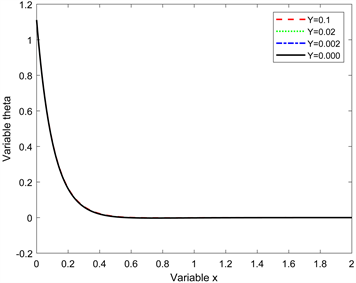(a)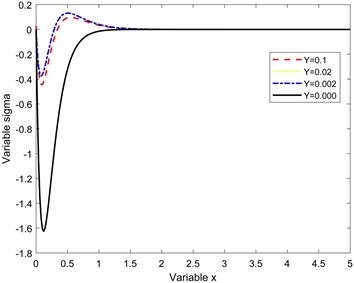(b)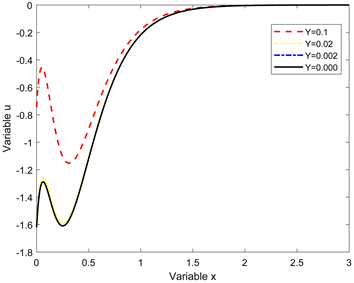(c)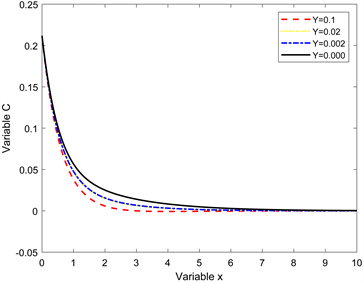(d)(e)

Figure 1. Influence trend of non-local parameters on various physical quantities. (a) Influence of non-local parameters on temperature; (b) Influence of non-local parameters on stress; (c) Influence law of non-local parameters on displacement; (d) Influence of non-local parameters on concentration; (e) Influence rule of non-local parameters on chemical potential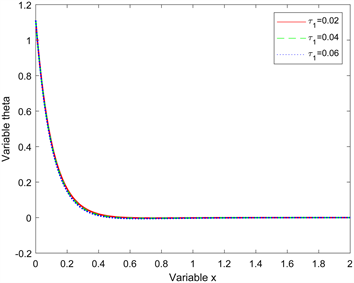(a)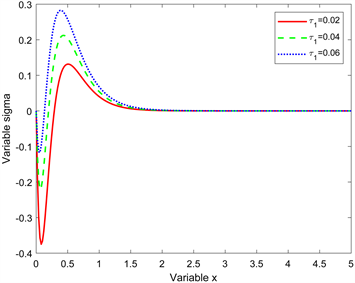(b)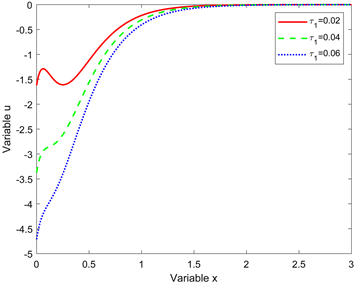(c)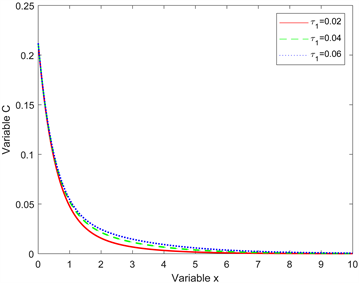(d)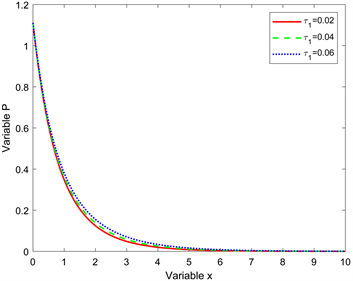(e)

Figure 2. Influence trend of heat conduction time relaxation factors on various physical quantities. (a) The influence of heat conduction time relaxation factor on temperature; (b) The influence of heat conduction time relaxation factor on stress; (c) The influence of heat conduction time relaxation factor on displacement; (d) The influence of heat conduction time relaxation factor on concentration; (e) The influence of heat conduction time relaxation factor on chemical potential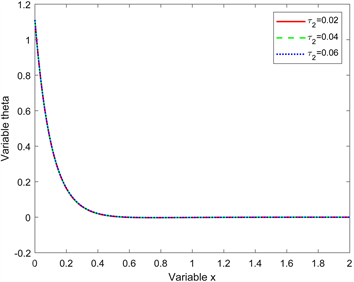(a)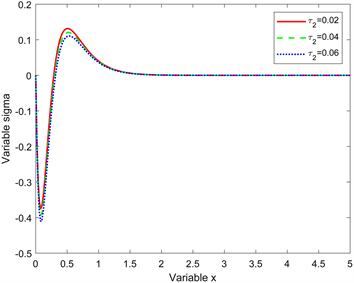(b)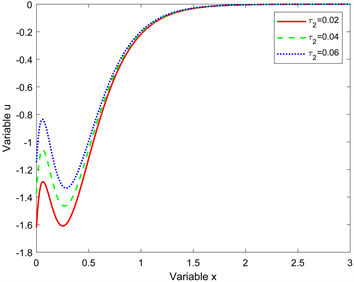(c)(d)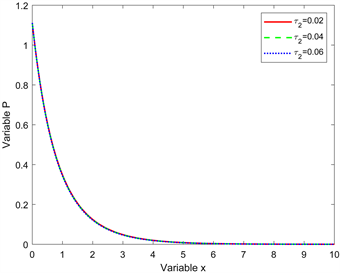(e)

Figure 3. Influence trend of diffusion time relaxation factor on various physical quantities. (a) The influence of diffusion time relaxation factor on temperature; (b) The influence of diffusion time relaxation factor on stress; (c) The influence of diffusion time relaxation factor on displacement; (d) The influence of diffusion time relaxation factor on concentration; (e) The influence of diffusion time relaxation factor on chemical potential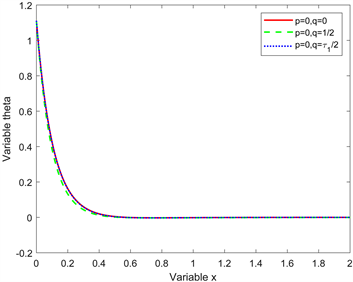(a)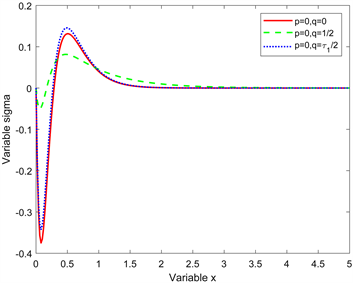(b)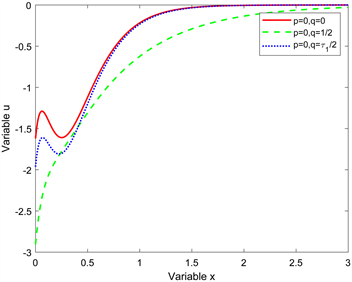(c)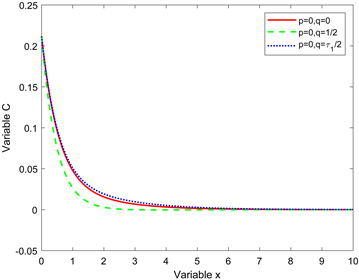(d)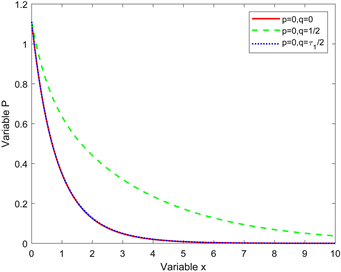(e)

Figure 4. Influence trend of kernel function on each physical quantity. (a) Influence rules of different kernel functions on temperature; (b) Influence rules of different kernel functions on stress; (c) Influence rules of different kernel functions on displacement; (d) Influence rules of different kernel functions on concentration; (e) Influence rules of different kernel functions on chemical potential

7. 结论

1) 非局部参数仅仅对和本构方程相关的物理量的影响较大，对温度、浓度和化学势几乎没有影响；

2) 不同的核函数对各个物理量都有不同的影响。这也验证了选取不同的核函数可以解决不同模型的问题，体现了它的灵活性和优越性；

3) 在非局部效应的影响下，热传导弛豫时间和扩散弛豫时间可以根据需要自由选择，由此可以得到更多的更为精确的力学行为。

  Lord, H.W. and Shulman, Y.A. (2007) A Generalized Dynamical Theory of Thermoelasticity. Journal of the Mechanics and Physics of Solids, 15, 299-309. https://doi.org/10.1016/0022-5096(67)90024-5  Green, A. and Lindsay, K.A. (1972) Thermoelasticity. Journal of Elasticity, 2, 1-7. https://doi.org/10.1007/BF00045689  Youssef, H.M. (2013) Theory of Two-Temperature-Generalized Ther-moelasticity. IMA Journal of Applied Mathematics, 71, 383-390. https://doi.org/10.1093/imamat/hxh101  Tzou, D.Y. (1995) A Unified Field Approach for Heat Conduction From Macro- to Micro-Scales. Journal of Heat Transfer, 117, 8-16. https://doi.org/10.1115/1.2822329  Qi, X. and Suh, C.S. (2010) Generalized Thermo-Elastodynamics for Semiconductor Material Subject to Ultrafast Laser Heating. Part I: Model Description and Validation. International Journal of Heat & Mass Transfer, 53, 41-47. https://doi.org/10.1016/j.ijheatmasstransfer.2009.10.010  Kuang, Z.B. (2009) Variational Principles for Ge-neralized Dynamical Theory of Thermopiezoelectricity. Acta Mechanica, 203, 1-11. https://doi.org/10.1007/s00707-008-0039-1  Wang, Y.Z., Zhang, X.B. and Song, X.N. (2014) A Generalized Theory of Thermoelasticity Based on Thermomass and Its Uniqueness Theorem. Acta Mechanica, 225, 797-808. https://doi.org/10.1007/s00707-013-1001-4  Li, C., Chen, Y. and Kurths, J. (2013) Fractional Calculus and Its Applications. Philosophical Transactions, 371, Article ID: 20130037. https://doi.org/10.1098/rsta.2013.0037  Liu, L.L. and Duan, J.S. (2015) A Detailed Analysis for the Funda-mental Solution of Fractional Vibration Equation. Open Mathematics, 13, 111-129. https://doi.org/10.1515/math-2015-0077  李岩. 分数阶微积分及其在粘弹性材料和控制理论中的应用[D]: [博士学位论文]. 济南: 山东大学, 2008.  Povstenko, Y.Z. (2012) Nonaxisymmetric Solutions of the Time-Fractional Heat Conduction Equation in a Half-Space in Cylindrical Coordinates. Journal of Mathematical Sciences, 183, 252-260. https://doi.org/10.1007/s10958-012-0811-6  Youssef, H.M. and Al-Lehaibi, E.A. (2010) Fractional Order Generalized Thermoelastic Half-Space Subjected to Ramp-Type Heating. Mechanics Research Communications, 37, 448-452. https://doi.org/10.1016/j.mechrescom.2010.06.003  Ef, S., et al. (2004) The Theory of Generalized Ther-moelastic Diffusion. International Journal of Engineering Science, 42, 591-608.  Sherief, H.H. and Saleh, H.A. (2005) A Half-Space Problem in the Theory of Generalized Thermoelastic Diffusion. International Journal of Solids & Structures, 42, 4484-4493. https://doi.org/10.1016/j.ijsolstr.2005.01.001  马永斌, 何天虎. 基于分数阶热弹性理论的含有球型空腔无限大体的热冲击动态响应[J]. 工程力学, 2016(7): 31-38.  何天虎, 李林. 分数阶热弹性理论下的广义电磁热弹问题[J]. 兰州理工大学学报, 2016, 42(2): 167-172.  Wang, J.L. and Li, H.F. (2011) Surpassing the Fractional Derivative: Concept of the Memory-Dependent Derivative. Computers & Mathematics with Applications, 62, 1562-1567. https://doi.org/10.1016/j.camwa.2011.04.028  Othman, M. and Sur, A. (2020) Transient Response in an Elasto-Thermo-Diffusive Medium in the Context of Memory-Dependent Heat Transfer. Waves in Random and Complex Media, 3, 1-24. https://doi.org/10.1080/17455030.2020.1737758  李妍. 广义热弹扩散记忆依赖问题的瞬态响应分析[D]: [硕士学位论文]. 兰州: 兰州理工大学, 2019.  Wilmanski, K. (1979) Localization Problem of Nonlocal Conti-nuum Theories. Archives of Mechanics, 85, 317-325.  Eringen and Cemal, A. (1983) On Differential Equations of Nonlocal Elasticity and Solutions of Screw Dislocation and Surface Waves. Journal of Applied Physics, 54, 4703-4710. https://doi.org/10.1063/1.332803